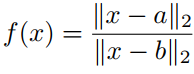# Minimizing perspective of a function

Hello,
I would like to minimize the function h(x, y)=\frac{x^n}{y^{n-1}} where x >0, y >0 and n is a positive integer. h(x, y) is the perspective of function f(x)=x^n, and hence, it is convex. For n=2, I have used the built-in function quad_over_lin. Is there any standard procedure for a general value of n?.

One way is to use gp mode via cvx_begin gp

minimize(x^n/y^(n-1))

1 Like

Assume you have the power cone

t^{1/n}y^{1-1/n} \geq |x|

constraint. That implies

t \geq \frac{|x|^n}{y^{n-1}}

so you could minimize x subject to a power cone constraint.

In Mosek v9 we will support the 3 dimensional power cone. For n=3 you can do it with conic quadratic optimization (warning of changed notation):

\begin{array}{c} \left \{(t,x,y)\mid t \geq \frac{|x|^3}{y^2}, y\geq 0 \right \} \\ \Leftrightarrow \\ \left \{(t,x,y)\mid (z,x) \in Kq, (\frac{y}{2},s,z), (\frac{t}{2},z,s) \in Kr \right \}. \end{array}

%
where Kq is a quadratic cone and Kr is a rotated quadratic cone.

I think it should also be possible for other n but has not worked out the details.

1 Like

Hi
this expression(named:distance ratio) is convexbut cvx don’t recognize it
Is any solution for reformulating it and solving?.

@alian Even in one dimension, that function is indefinite, i.e., neither convex nor concave.

If you believe I’m wrong, show us your proof of convexity.

Mark is correct. That is not convex.

yes . In general not convex but if we accept a constraint then is convex
proof

Convex Optimization[Boyd]

You are misreading that text. There are no circumstances where the ratio is convex. Regardless, CVX will not accept it; please read our FAQ.

Quasi-convex is not the same as convex.

Perhaps you can use the bisection method, described in section 4.2.5 of that book, presuming that you can produce an interval known to contain the solution to your problem, and contained within the quasiconvex halfspace. In such case, you might be able to use CVX to solve feasibility problems arising in the bisection.

yes, you are right. I write it in another form.